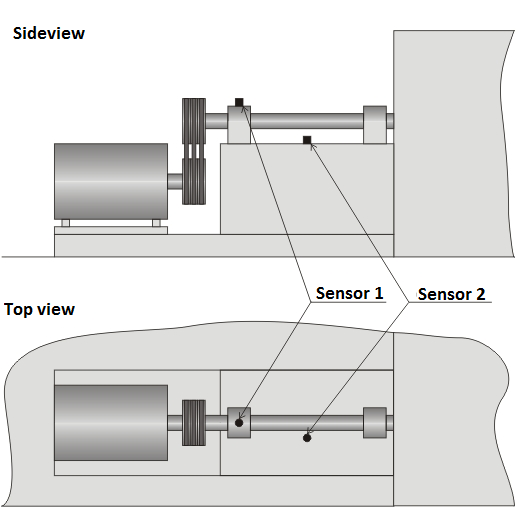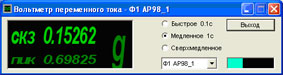# Vibrational measurement at the food plant

The following description of measurements took place at one of the near Moscow bread-baking plant. The purpose of the measurements was to determine the vibrational levels during bearing units operation of the fan-driven joint (see Figures 1 and 2).Figure 1Figure 2

The measurements were carried out using ZET 017-U2 АР98, personal computer of the Notebook type and two vibration transducers AP98.

For a full-scale diagnostic of the bearing units it was necessary to conduct the measurements in several stages at every stage the sensors were situated at different control points (see Figures 3 to 8).Figure 3Figure 4Figure 5Figure 6Figure 7Figure 8

For the real time, vibration parameters measurement was utilized such software of ZETLab as AC voltmeter, Vibrometer and Fractional octave spectrum analysis. In order to obtain the values of vibration velocity and vibration displacement out of vibration acceleration with the help of the 1-st and 2-nd order integration, the “Signal filtration” program was used. The signal filtration program was also used for creating the low and high frequencies from 10 Hz up to 1 kHz in accordance with primary positions of vibration parameters measurement under the State Standard ISO 2954-75. At the same time the signal recording were conducted using “Recorder (file signal recording)” program.

The results of the 2-nd stage measurements (the most informative and the one with more complete evaluation of the vibration parameters) are shown below. Sensor 1 (measuring channel 1) is mounted on the 1-st bearing unit in vertical position. Sensor 2 (measuring channel 2) is mounted on the 2-nd bearing unit in vertical position (see Figure 5).

Vibration acceleration at 1-st and 2-nd spot are shown in Figures 9 and 10 respectively:Figure 9Figure 10

Vibration velocity at 1-st and 2-nd spot are shown in Figures 11 and 12 respectively:Figure 11Figure 12

Vibration displacement at 1-st and 2-nd spot are shown in Figures 13 and 14 respectively:Figure 13Figure 14

Evaluation of the general integral level of vibration (dB) was carried out in 1/3-octave spectral bands using the linear correction of amplitude-frequency characteristic (AFC). The integral level indicated by the green scale.

Vibration acceleration of 1/3-octave spectra at 1-st and 2-nd spot are shown in Figures 15 and 16 respectively:Figure 15Figure 16

Vibration velocity of 1/3-octave spectra at 1-st and 2-nd spot are shown in Figures 17 and 18 respectively:Figure 17Figure 18

1/3-octave spectra vibration displacement at 1-st and 2-nd spot are shown in Figures 19 and 20 respectively:Figure 19Figure 20

In accordance with requirements of State Standard IEC 34-14-82 the average quadratic value of the vibration velocity is the criteria for the vibration severity evaluation.

For the regular engine types with rated rotational speed from 600 up to 6,000 rpm ultimate average quadratic value of the vibration velocity is 2.8 mm/s.

In our case these values are 1.99965 and 3.11904 mm/s for the 1-st and the 2-nd bearing units respectively.

General integral vibration levels (average quadratic value) of the vibration acceleration are 74.3 and 78.1 dB for the 1-st and the 2-nd bearing units respectively.

Delivery terms: CIP, CPT or DAP. Customs clearance and delivery is carried out by the "Cordely" custom company (included in the price of the goods). 1992 - 2023 ZETLAB Company